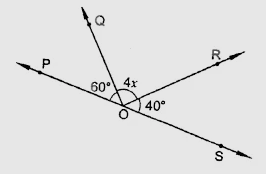"
">

# In the figure, POS is a line, find $x$."

Given:

POS is a line.

To do:

We have to find the value of $x$.

Solution:

We know that,

Sum of the angles on a straight line is $180^o$.

Therefore,

From the figure,

$\angle POQ + \angle QOR + \angle ROS = 180^o$

$60^o + 4x^o + 60^o = 180^o$

$4x = 180^o - 120^o$

$4x= 60^o$

$x = 15^o$

The value of $x$ is $15^o$.

Updated on: 10-Oct-2022

21 Views